# C++:C++11 和 设计模式"不如握紧拳头,这就是我的所有"

# 前言:C++11简介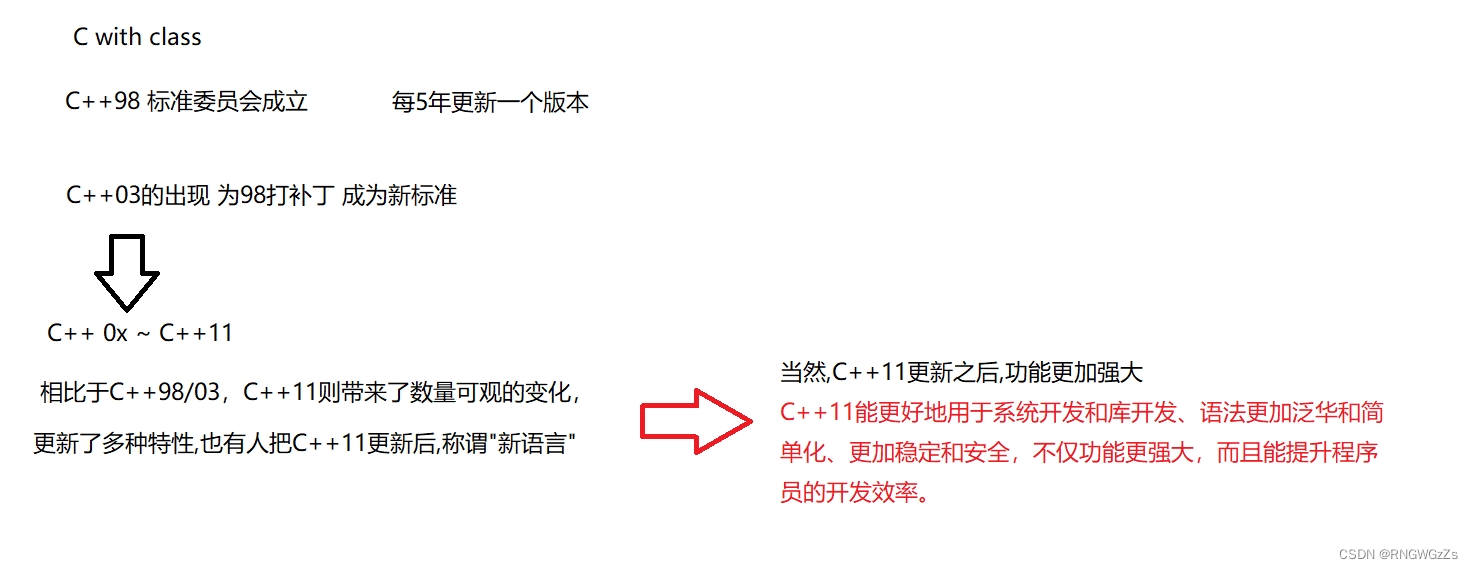# (一)C++11特性与语法

## (1)列表初始化

C++11对于 {} 初始化值赋予新的用法:

### ①内置类型+结构体

``````struct Point
{
int _x;
int _y;
};

int main()
{
//1结构体
Point p1{ 1,2 };
Point p2={ 3,4 };

//内置类型
int x{ 0 };
int y = { 1 };

return 0;
}``````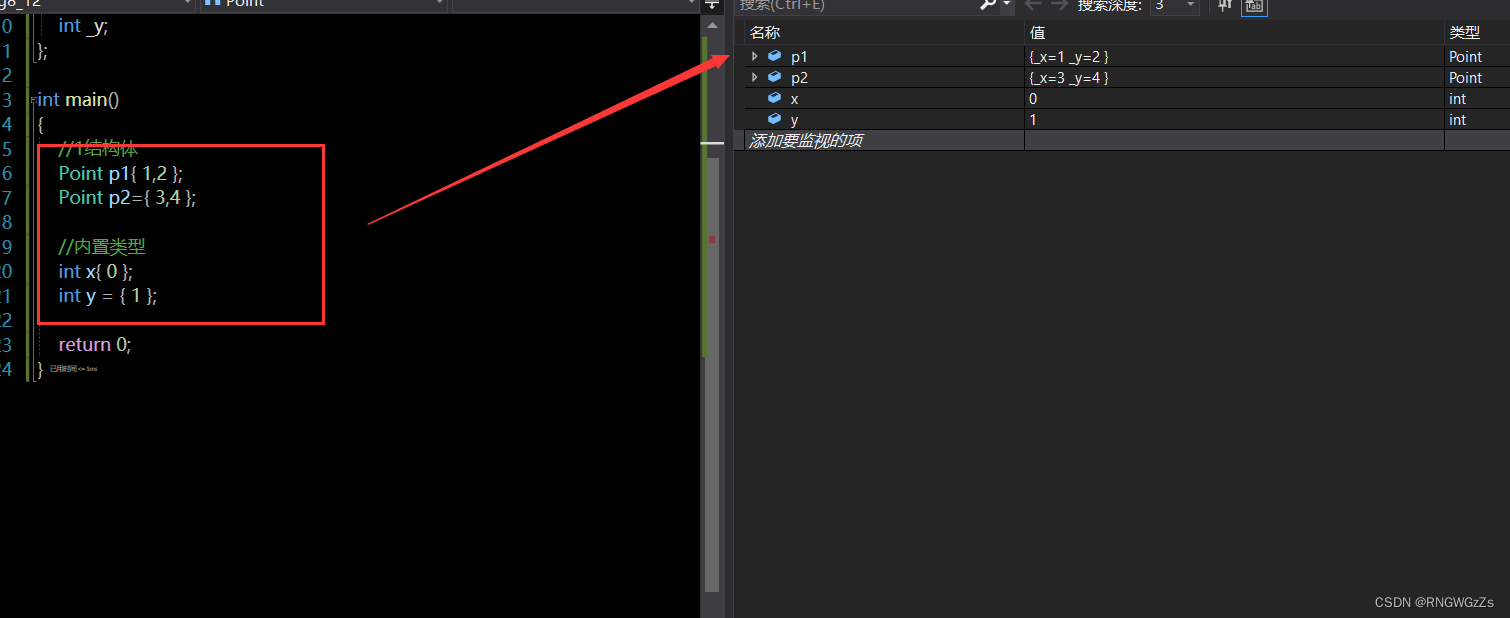但建议这些内置类型初始化还是采用 原先已有的方式处理.因为本来这个特性并不是为了这样初始化而设立的。

### ②vector\list\map 与 自定义类型

``````class Date
{
public:
Date(int year = 0, int month = 1, int day = 1)
:_year(year)
, _month(month)
, _day(day)
{
cout << "Date(int year, int month, int day)" << endl;
}

private:
int _year;
int _month;
int _day;
};

int main()
{
Date d1(2022, 11, 1);
Date d2={ 2022,11,15 };
Date d3{ 2022,11,18 };

vector<int> v1{ 1,2,3,4,5 };
//v1.push_back(1);
list<int> ls1{ 1,2,3,4 };
map<string, int> mp{ make_pair("insert",1),{"select",2} };
}
``````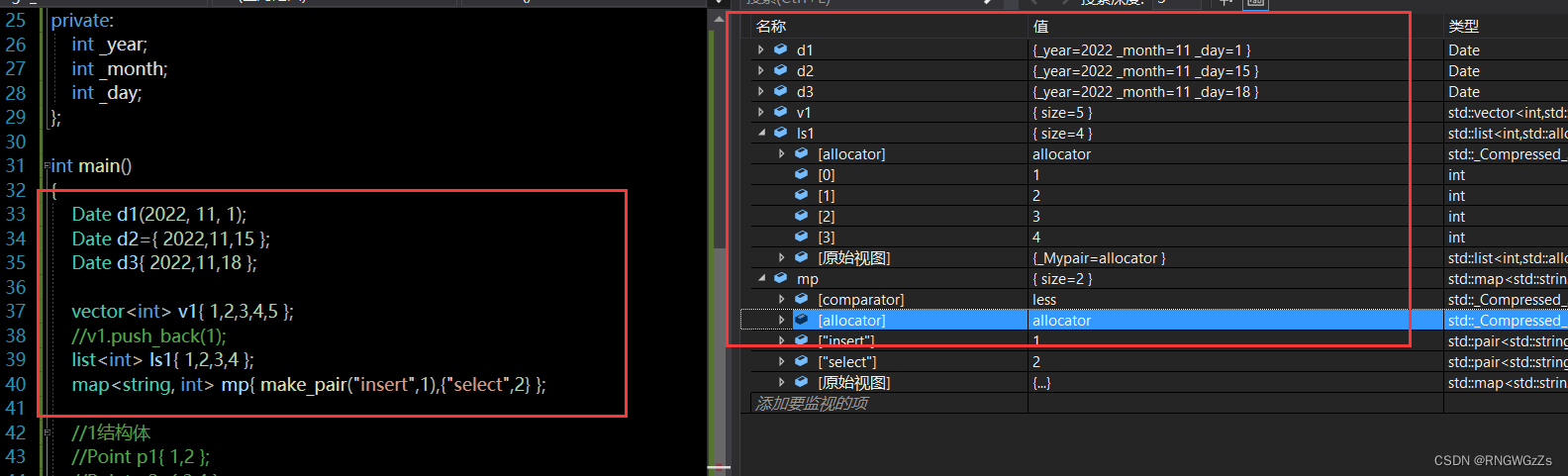### ③initializer_list

为什么这些容器支持这样的初始化,本质上是因为加入了新的模板类型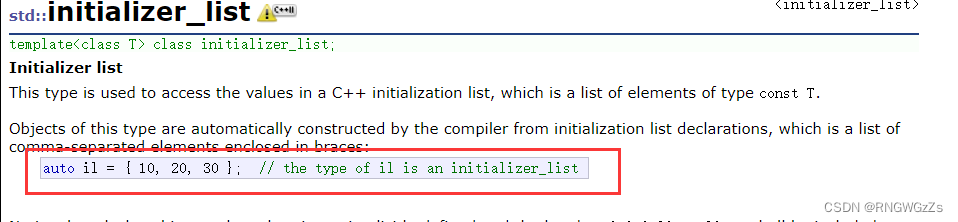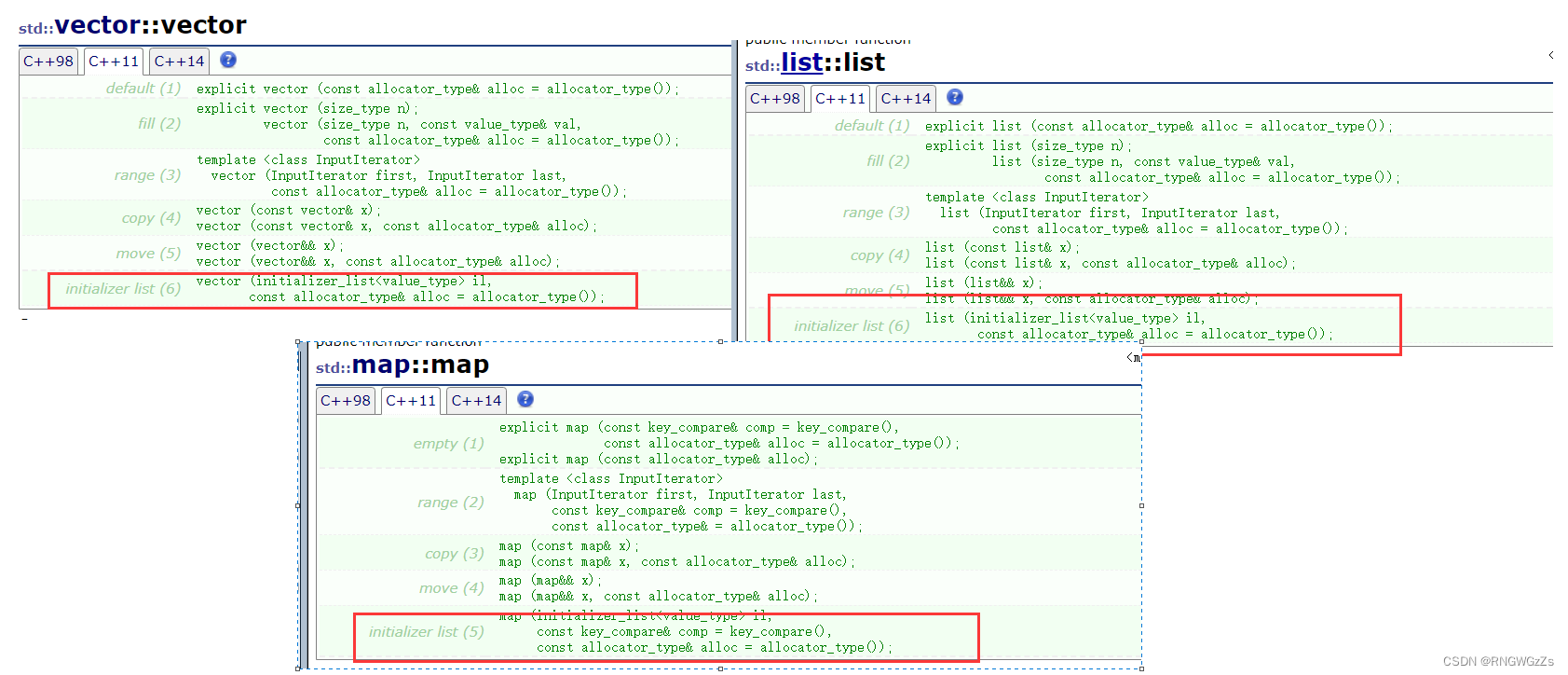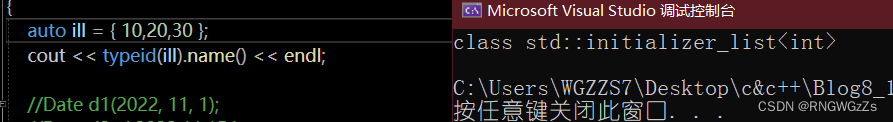## (2)变量声明

### ①decltype: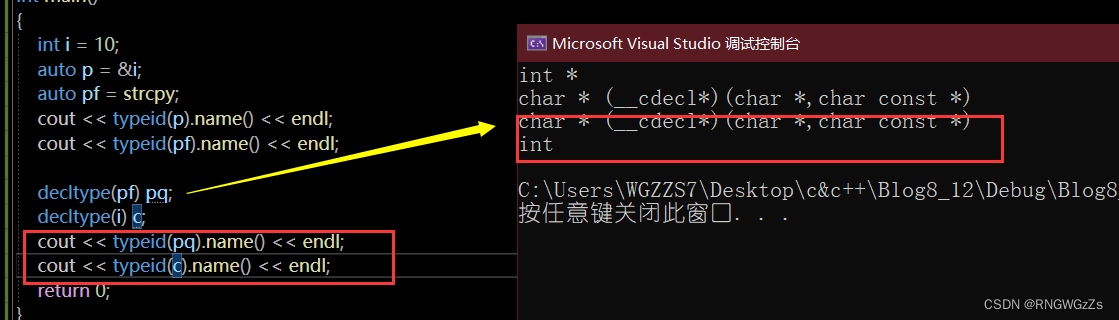本质上,decltype声明的类型,可以又作为类型去定义变量！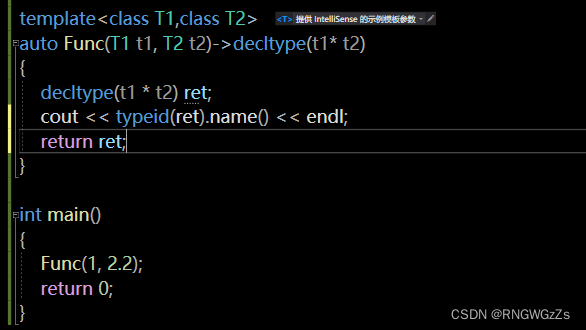## (3)范围for+ final/overrid;

final/overrid:一个是类不能被继承  一个是检查子类虚函数是否重写

### 注:NULL 与 nullptr的区别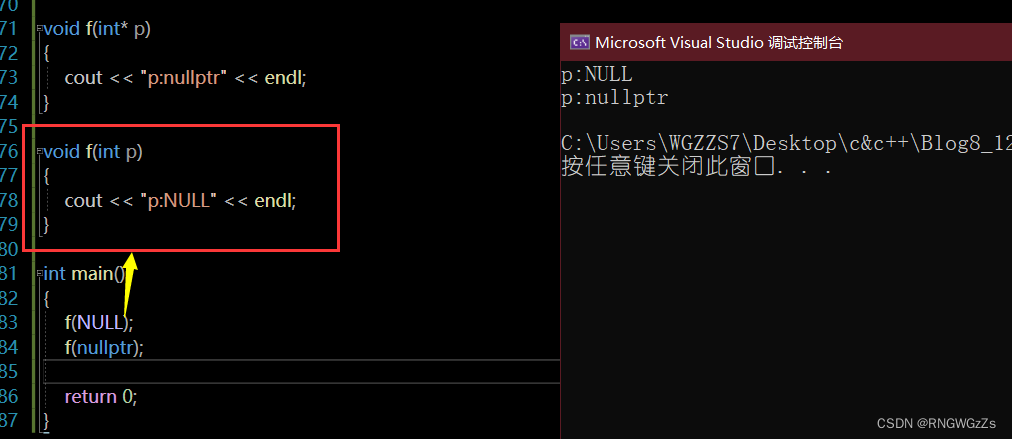对NULL而言,会把它认定整型,因为底层就是define 宏定义的 0。

## (4)STL新增容器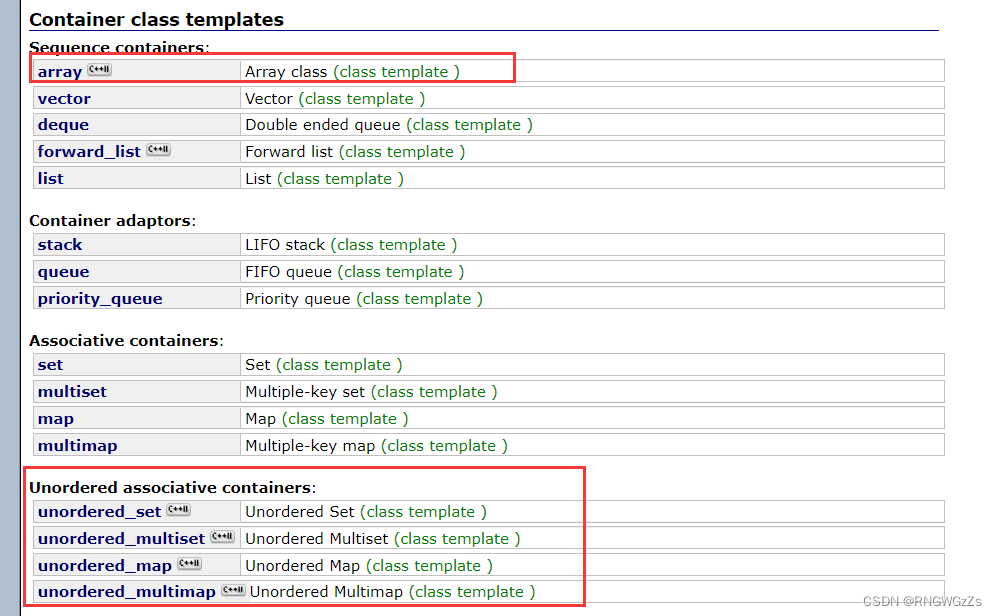C++11新增了几个容器,当然最重要的就是unordered_map 、unordered_set.

### ①array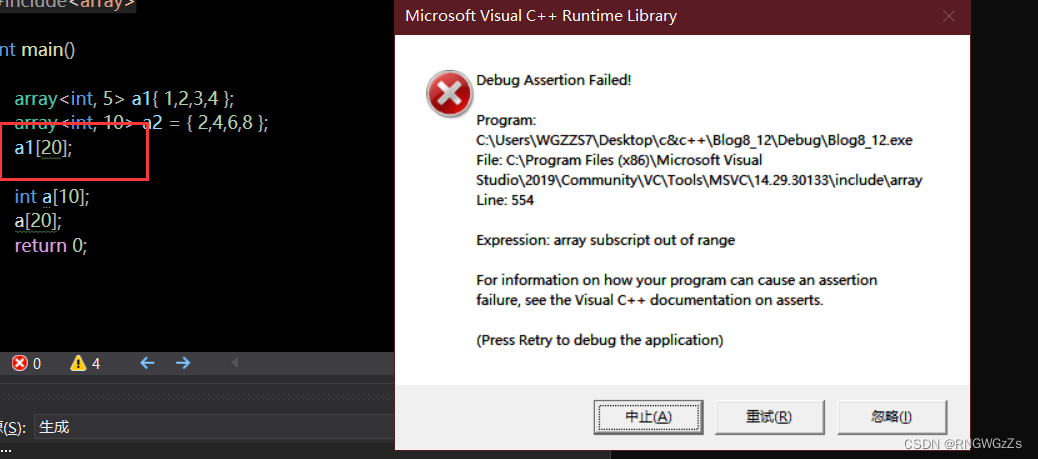## (5)左值引用与右值引用!!!(重点)

### ①什么是左值？

1.可以取地址&.

2.一般情况下可以进行修改(const为例外)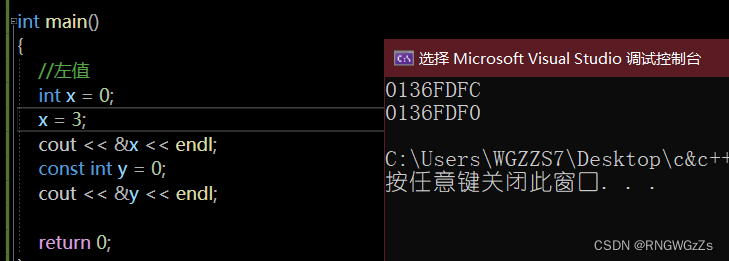### ②什么是右值?

1.不能修改

2.不能取地址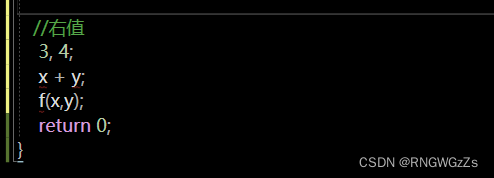### ③左右值引用;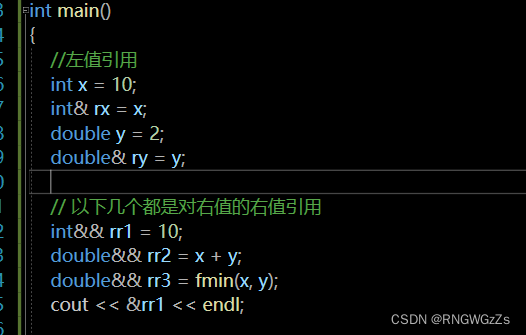### ④左右值 与 左右值引用的关系:

左值是否能被右值引用？ 右值是否能被左值引用？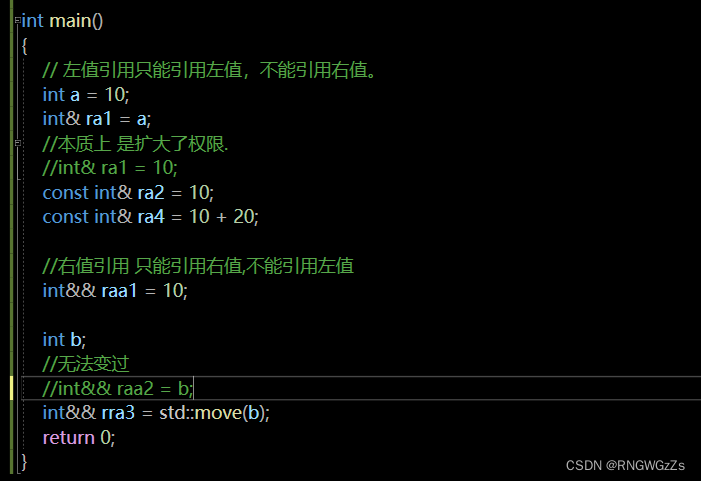const 左值引用 ------> 左值+右值

## (6)右值引用与移动语义(重点!!)

### ①左值引用的使用场景;

1.做函数参数

2.做返回值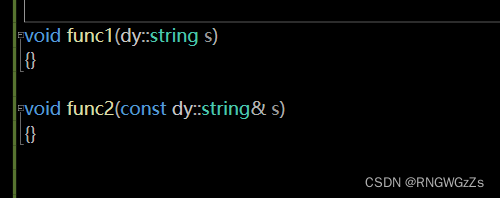### ②右值引用的价值和场景在这种情况下,左值无法做到减少拷贝的效果。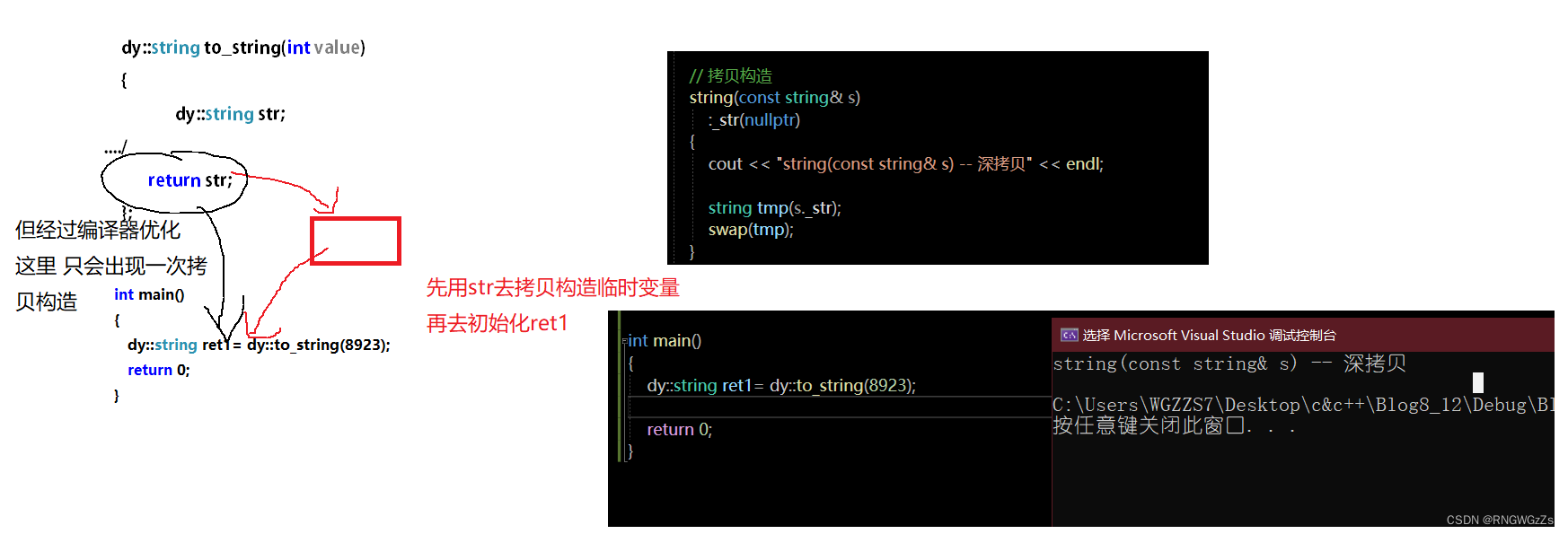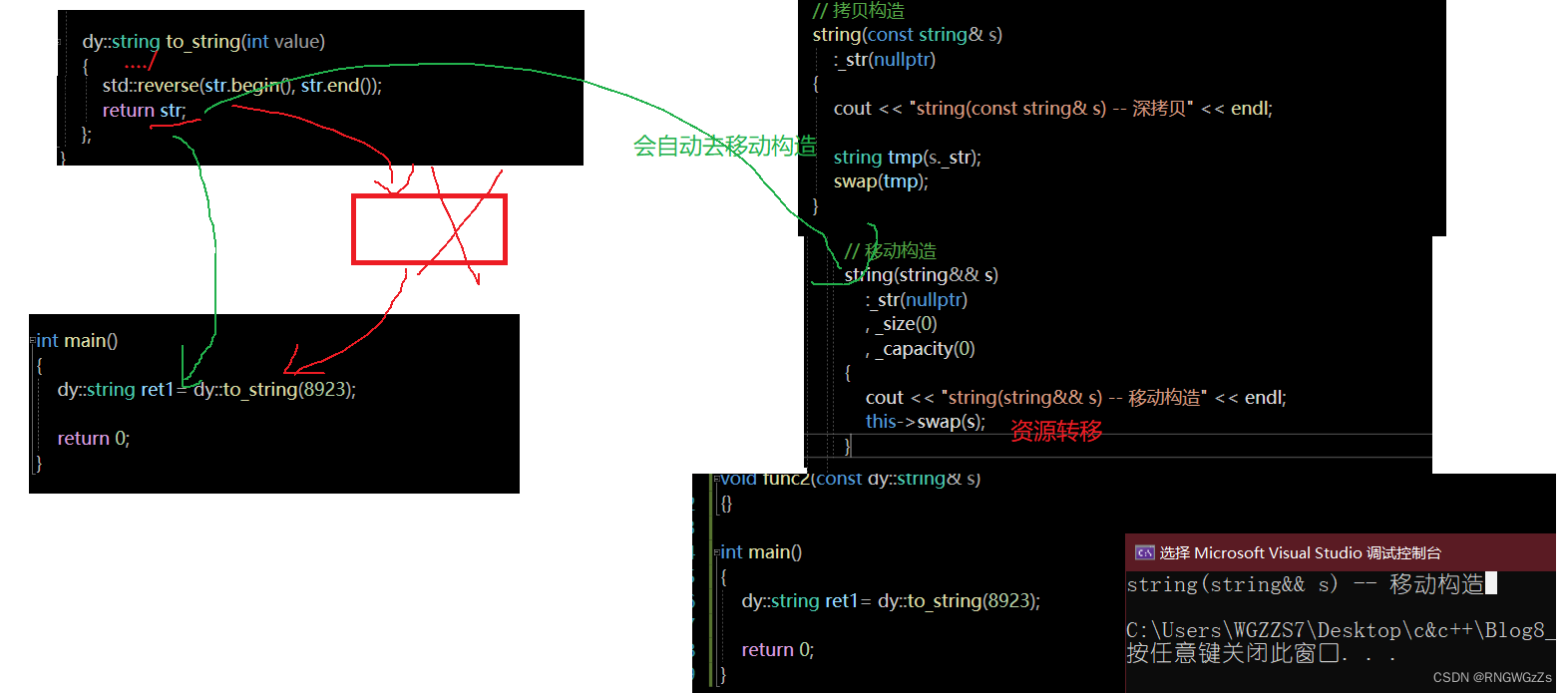移动赋值和移动构造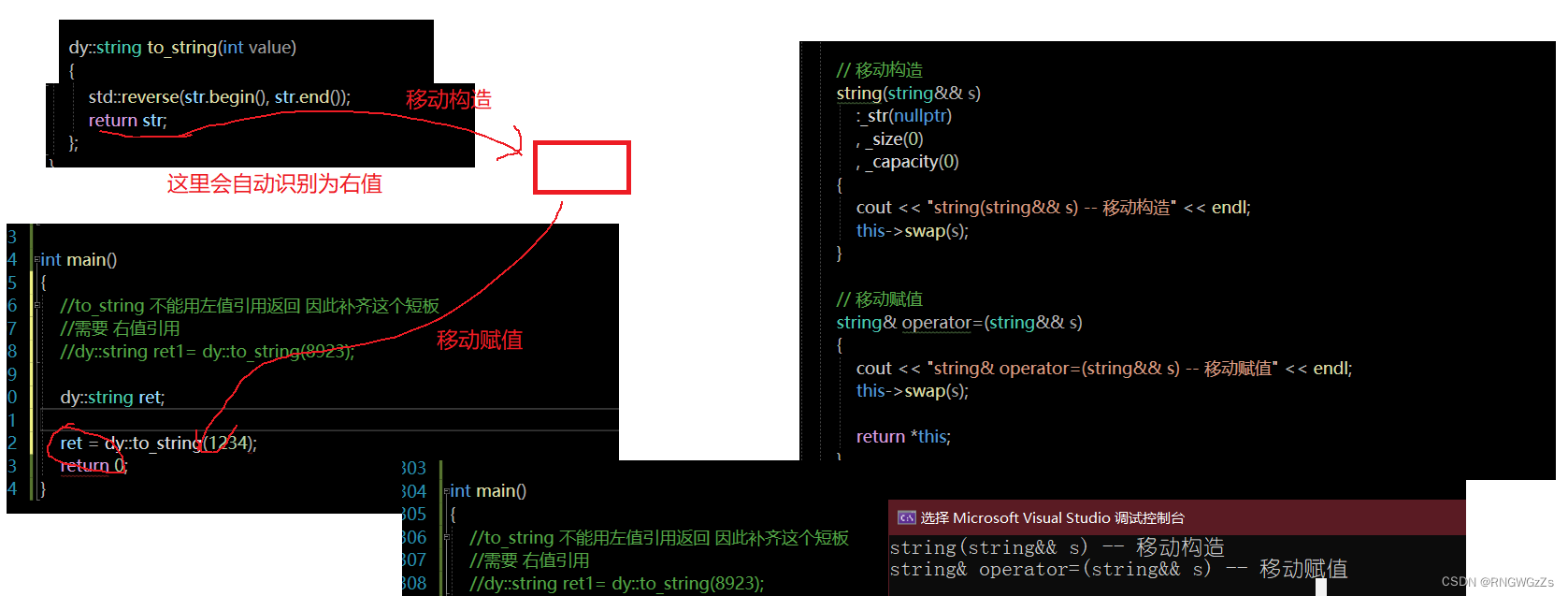### 其他右值引用使用场景: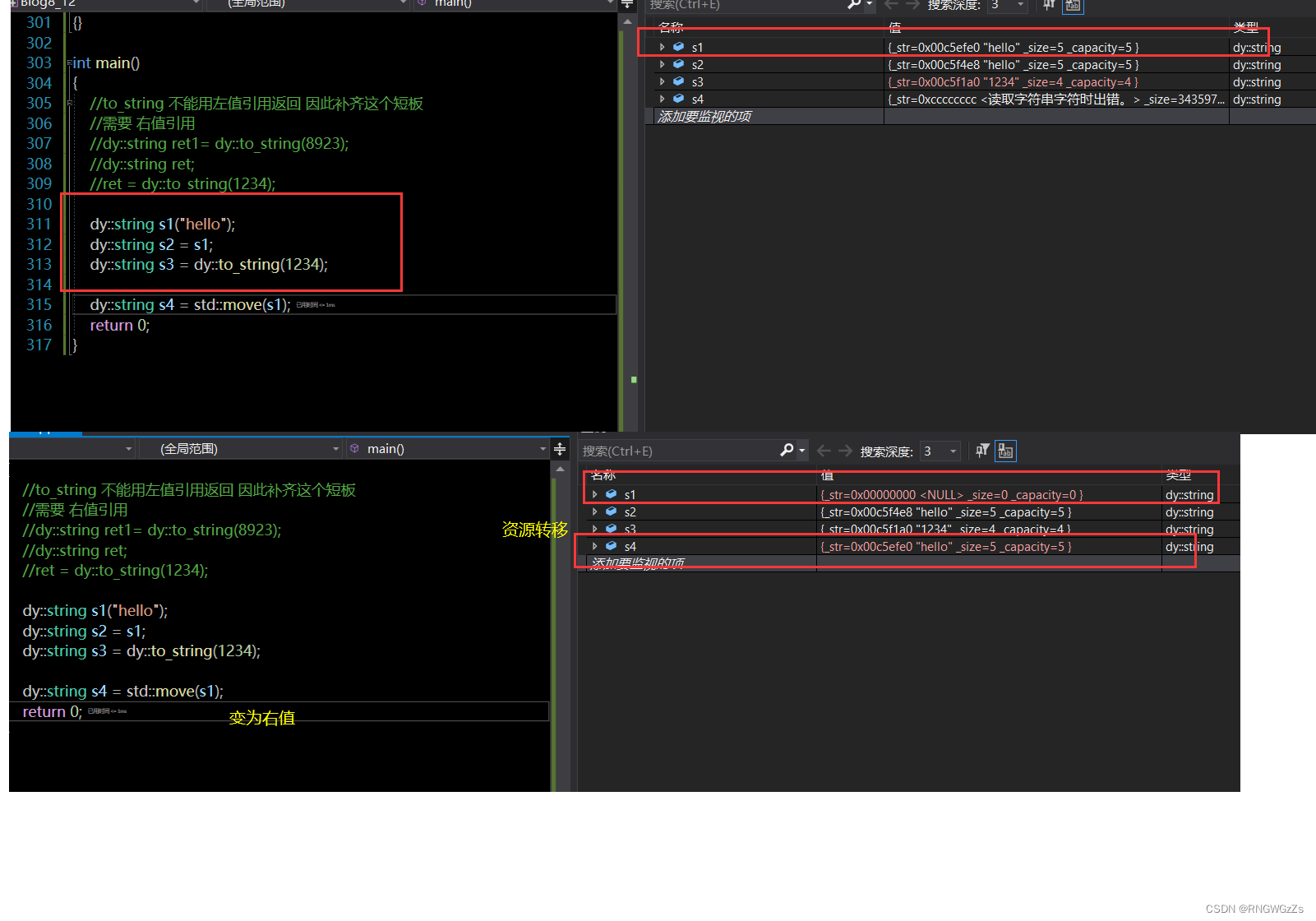进行std::move(左值)时,需要时刻谨慎。

## (7)万能引用+完美转发

### ①万能引用

template<class T>

void Test(T&& t)  //只要是模板+&& 就是万能引用

反之 内置类型+&& 就是右值引用

``````void Fun(int& x) { cout << "左值引用" << endl; }
void Fun(const int& x) { cout << "const 左值引用" << endl; }

void Fun(int&& x) { cout << "右值引用" << endl; }
void Fun(const int&& x) { cout << "const 右值引用" << endl; }

template<typename T>
void PerfectForward(T&& t)
{
Fun(std::forward<T>(t));
}

int main()
{
PerfectForward(10);           // 右值

int a;
PerfectForward(a);            // 左值
PerfectForward(std::move(a)); // 右值

const int b = 8;
PerfectForward(b);		      // const 左值
PerfectForward(std::move(b)); // const 右值

return 0;
}``````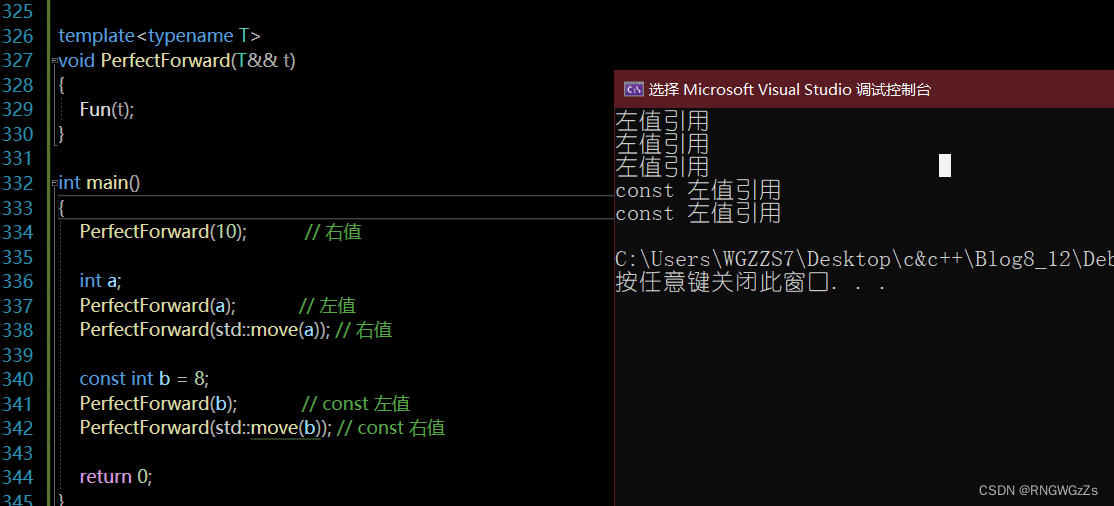1.模板的万能引用只是提供了能够接收同时接收左值引用和右值引用的能力.

2.但是引用类型的唯一作用就是限制了接收的类型，后续使用中都退化成了左值。

### ②完美转发

std::forward<T>(x);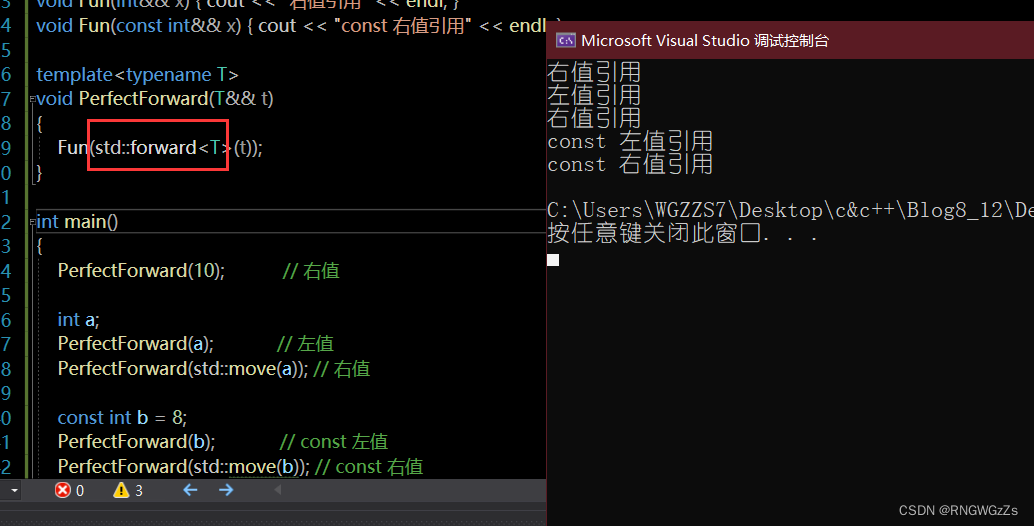## (8) 类的新功能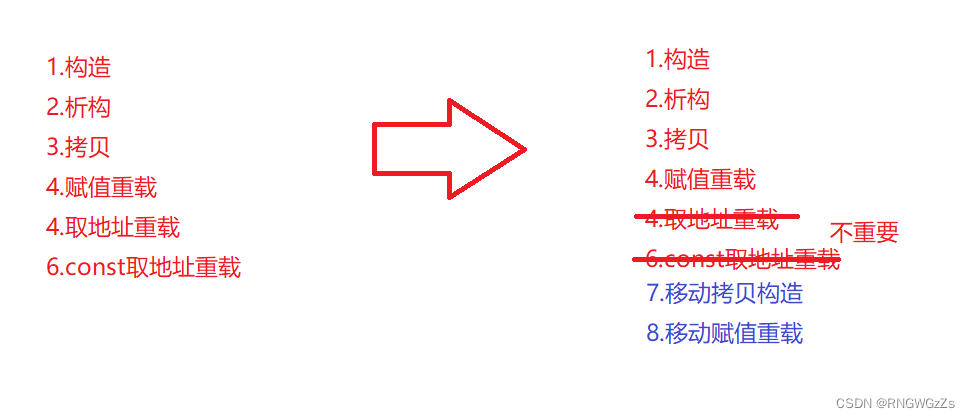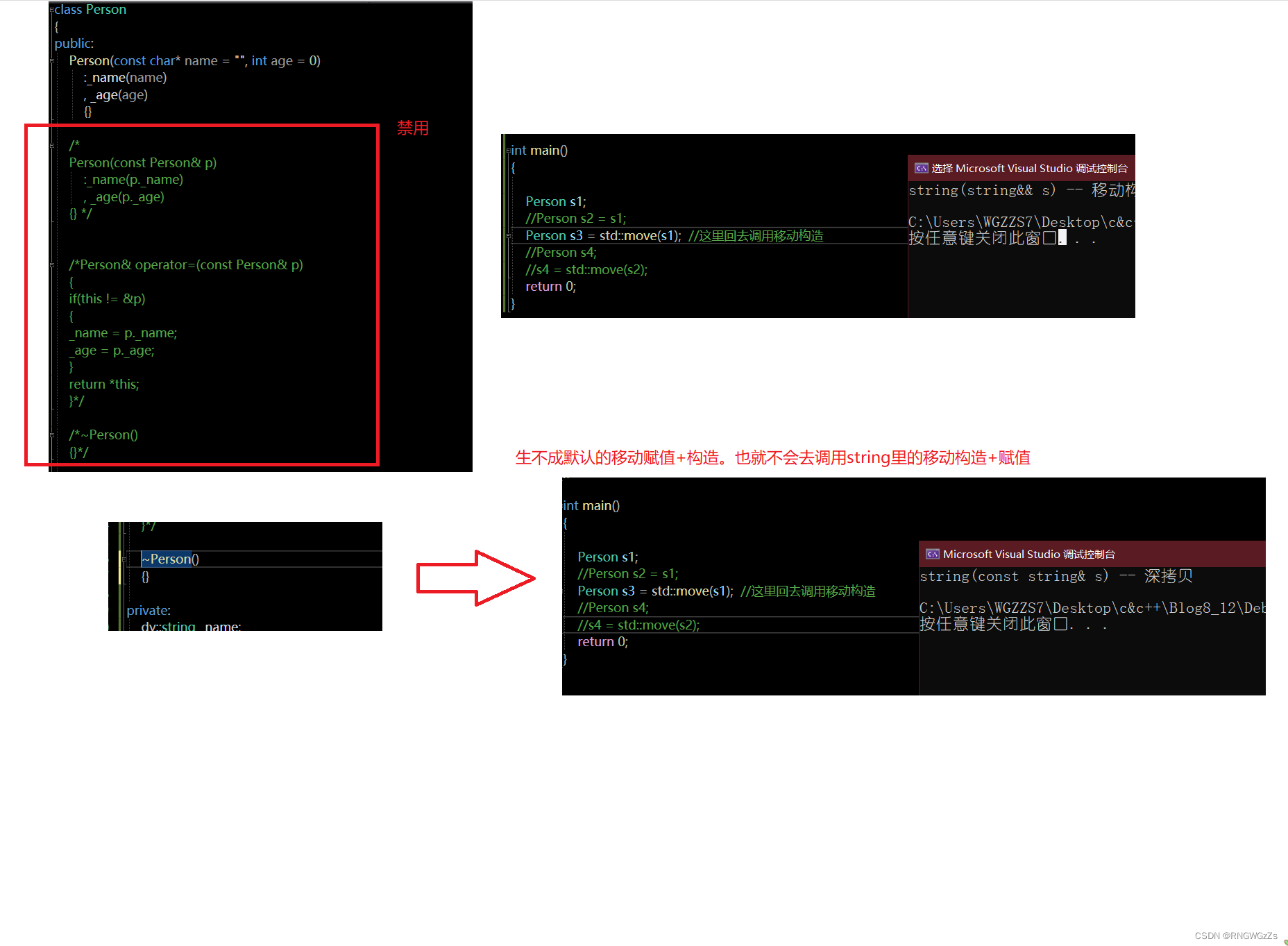### ①显式缺省函数

C++中也增加了两个关键字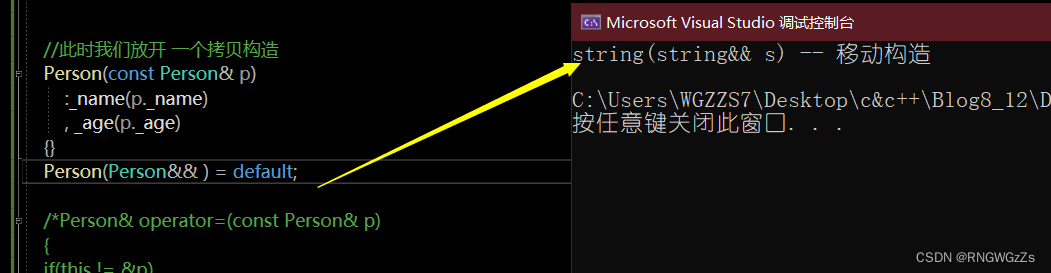### ②删除默认函数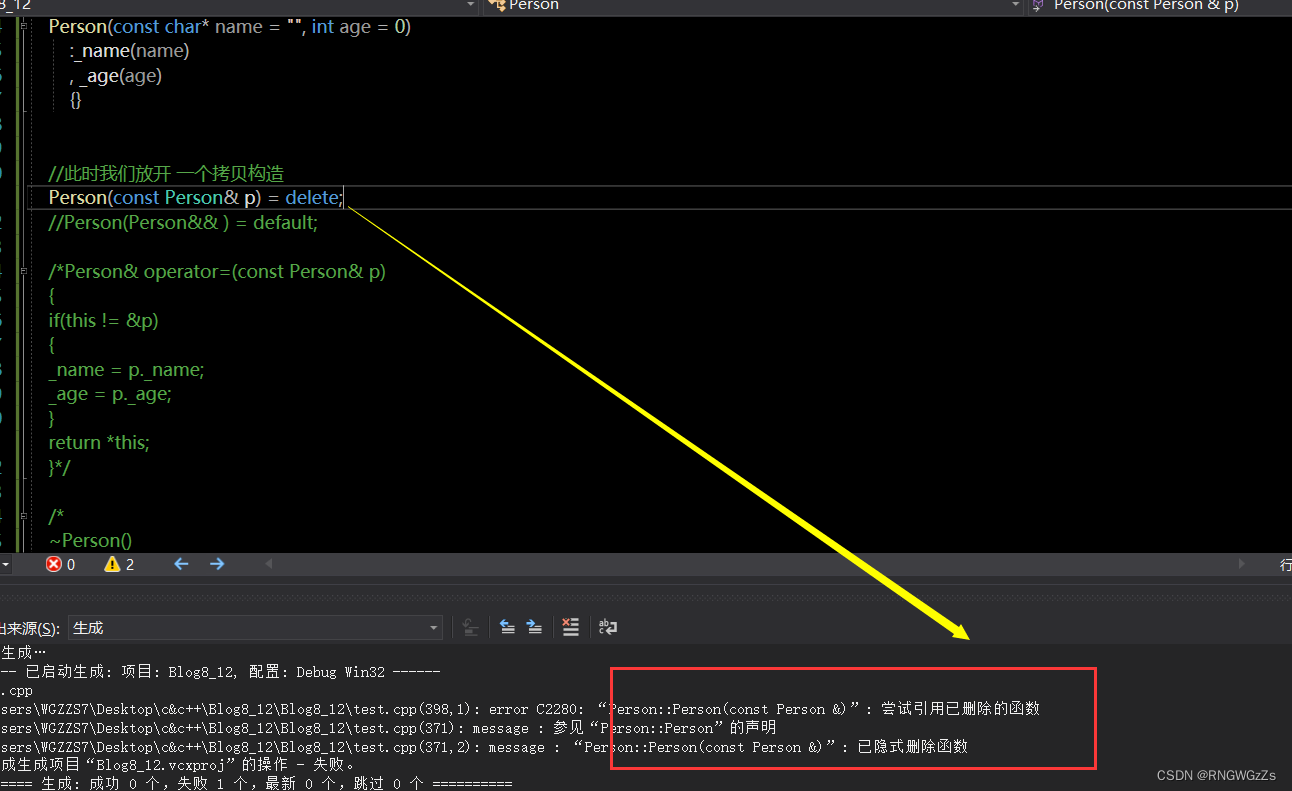## (9)可变参数模板

### ①什么是可变参数?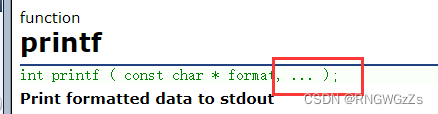### ②形式

template<class ...Args>

void ShowList(Args ...args)

{}

Args:模板参数包

args:一个形参的函数参数包

Args ...args:这个声明表示,可以有0~任意个模板参数

``````//计算 函数包
cout << sizeof...(args) << endl;``````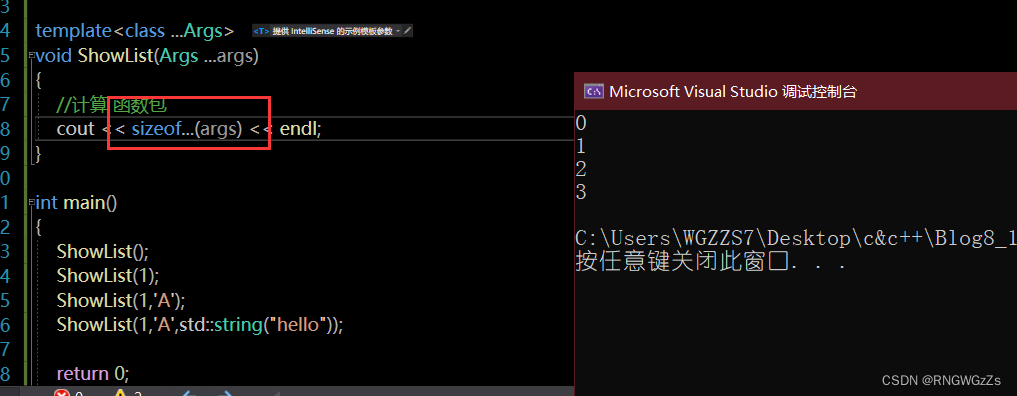### ③打印模板参数

``````//这个函数 主要是 为了读取args为空包的时候
void ShowList()
{
cout << endl;
}

template<class T,class ...Args>
void ShowList(T value,Args ...args)
{
//计算 函数包
/*cout << sizeof...(args) << endl;*/
//递归取出 参数包的内容
cout << value << " ";

//每次少一个参数
ShowList( args ...);
}

int main()
{
ShowList();
ShowList(1);
ShowList(1,'A');
ShowList(1,'A',std::string("hello"));

return 0;
}
``````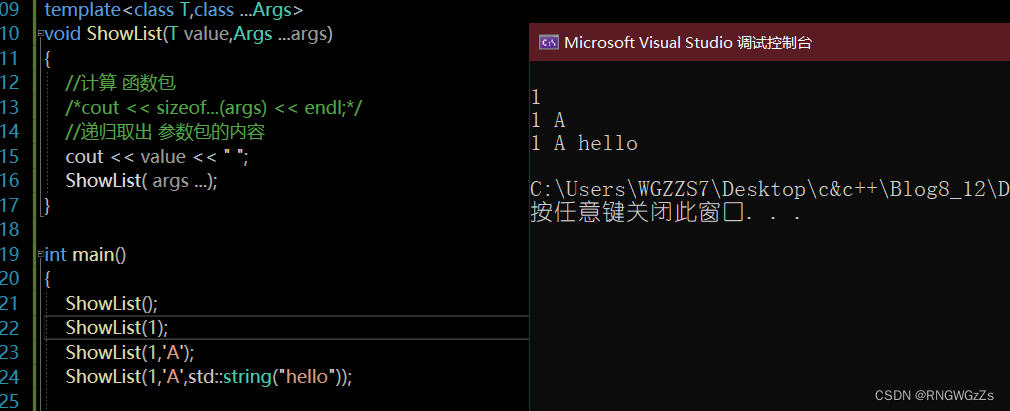注:但可变参数模板不支持 [] 去取参数包里的参数！！！

``````template<class ...Args>
void ShowList(Args ...args)
{
//列表初始化
int arr[] = { args... };
cout << endl;
}

int main()
{
//int 数组 传整形
ShowList(1, 2, 3, 4, 5);
//非整型则不能

return 0;
}``````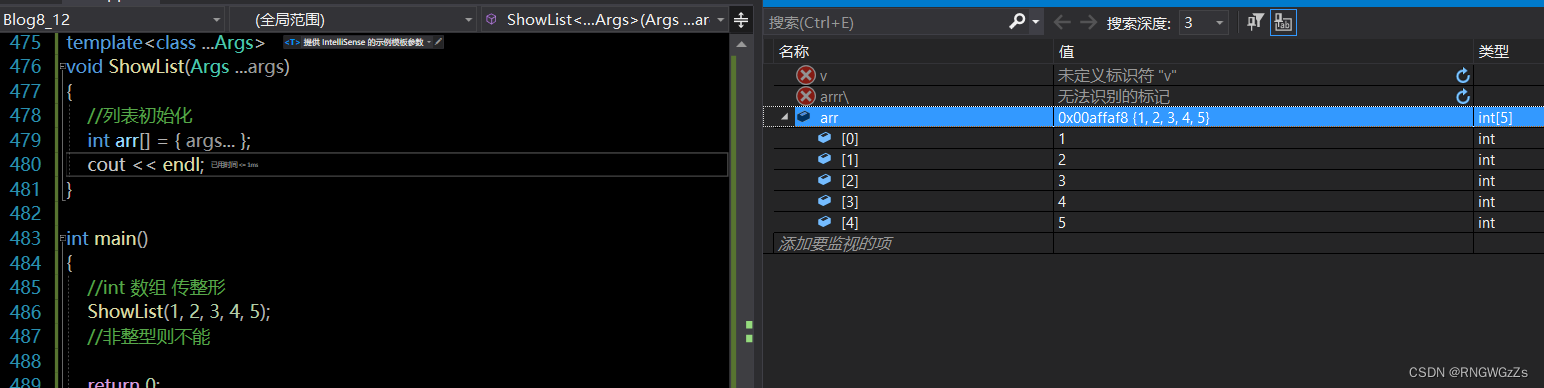``````void PrintArg(T t)
{
cout << t << " ";
}

template<class ...Args>
void ShowList(Args ...args)
{
//列表初始化
int arr[] = {(PrintArg(args),0)...};
cout << endl;
}

int main()
{
//int 数组 传整形
ShowList(1, 2, 3, 4, 5);
//非整型则不能
ShowList(1, "21312", 'A');
return 0;
}``````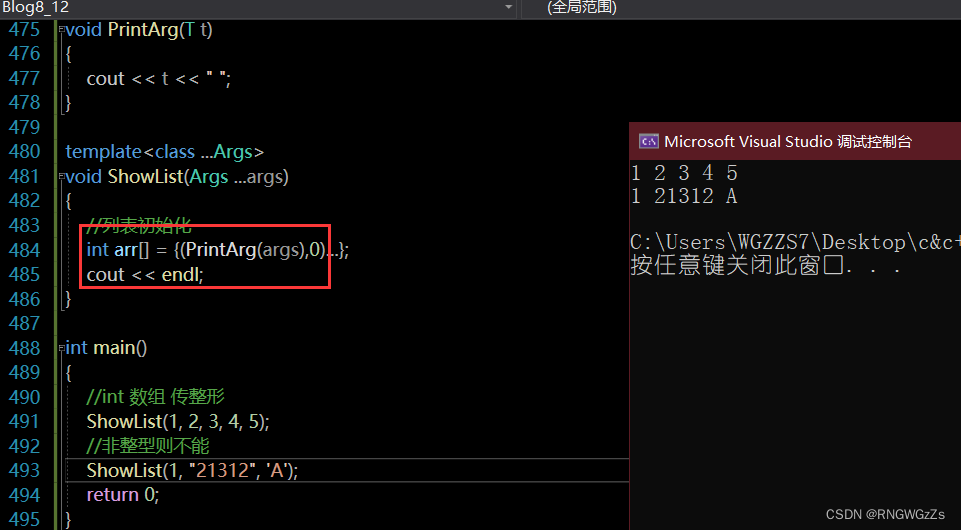### ④STL库中的emplace_系列:万能引用 + 可变参数模板emplace 与 push_back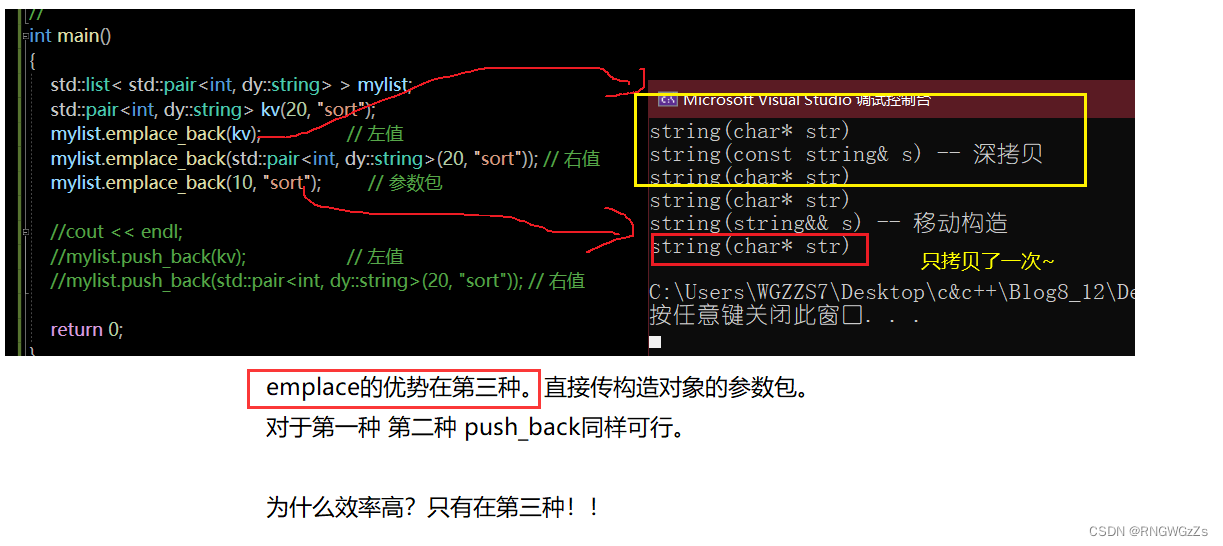## (10)lambda表达式

### ①语法

lambda表达式书写格式：

[capture-list] (parameters) mutable -> return-type { statement }

[捕捉列表]         (参数)               返回类型                        函数实现

[var]：表示值传递方式捕捉变量var

[=]：表示值传递方式捕获所有 父作用域中的变量 (成员函数中包括this)
[&var]：表示引用传递捕捉变量var
[&]：表示引用传递捕捉所有父作用域中的变量(包括this)

mutable ---> (可以更改)[a,b]  否则const不能更改。
[&a,&b] 则不具有const属性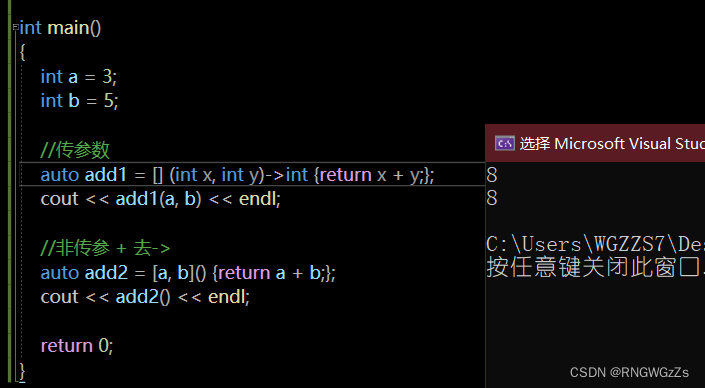### ②仿函数与lambda

``````struct Goods
{
string _name;
double _price;
int _num;

// ...
};

struct Com_num
{
bool operator()(const Goods& g1, const Goods& g2)
{
return g1._num > g2._num;  //降序数量
}
};
#include<algorithm>

int main()
{
vector<Goods> v = { { "苹果", 2.1, 300 }, { "香蕉", 3.3, 100 }, { "橙子", 2.2, 1000 }, { "菠萝", 1.5, 1 } };
sort(v.begin(), v.end(), Com_num());
//lambda表达式
sort(v.begin(), v.end(), [](const Goods& g1, const Goods& g2)
{
return g1._num < g2._num; //升序
});
}``````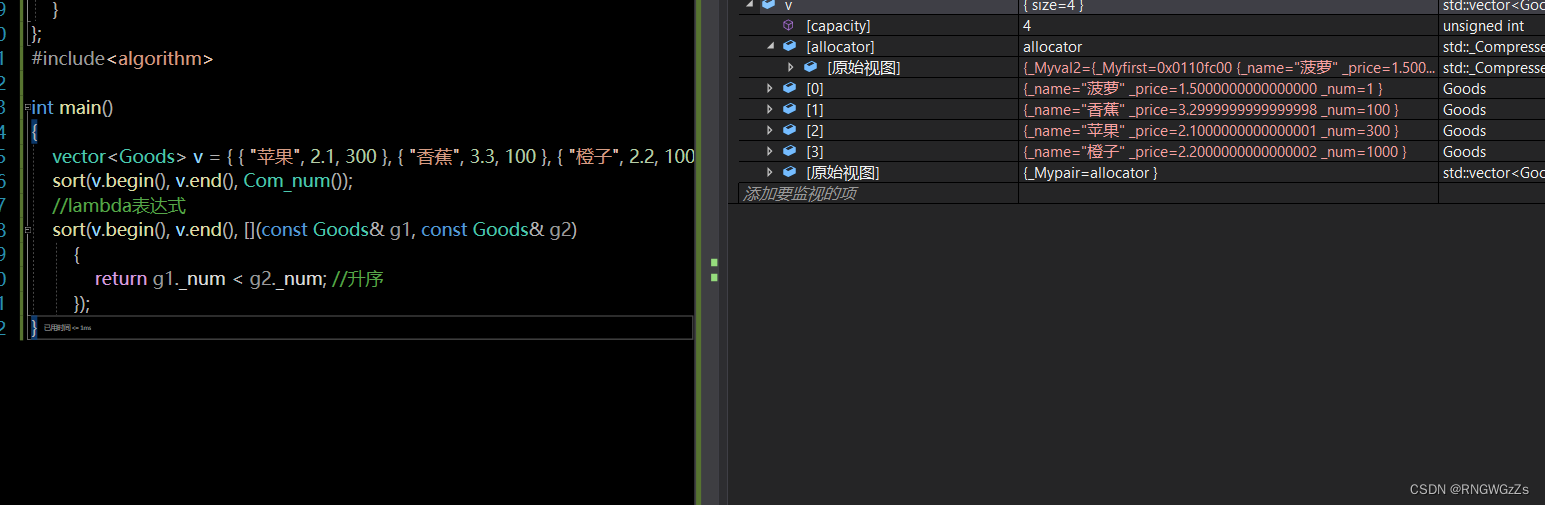lambda底层会被编译器转为仿函数(lambda+string(uuid)) 类的名称;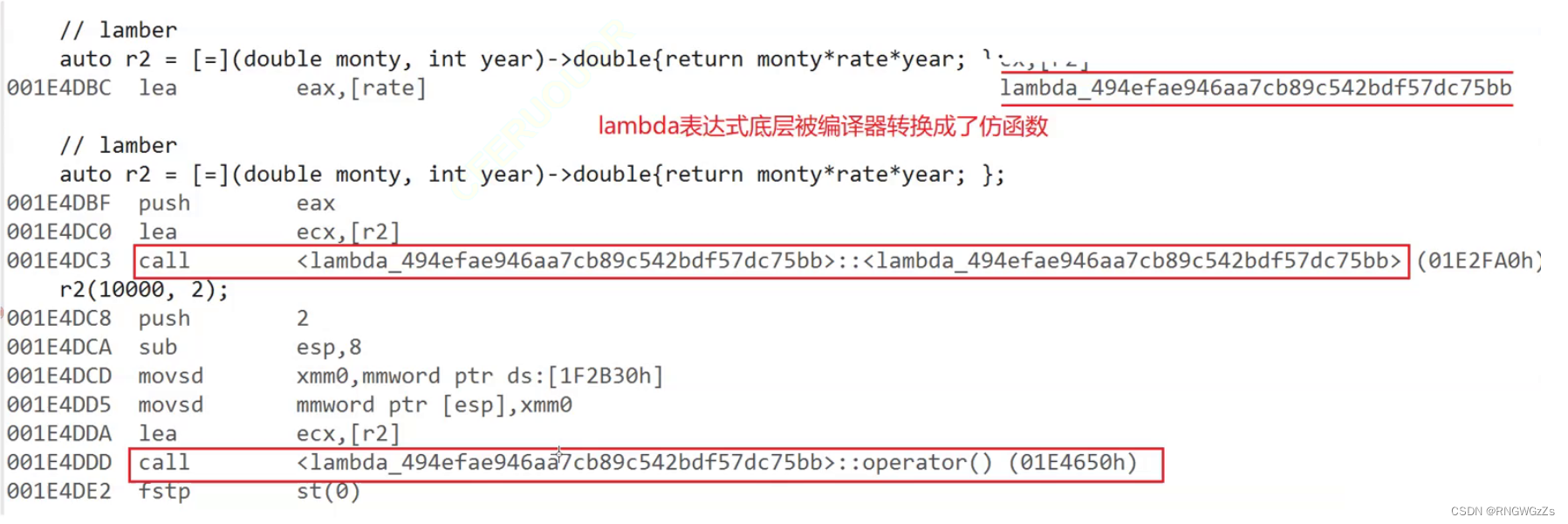## (11)包装器

std::function  头文件<functional>

template<class T> function;

template<class Ret,class ...Args>

class function<Ret(Args...)>;

Ret:返回值

Args:函数调用形参包

``````template<class F, class T>
T useF(F f, T x)
{
static int count = 0;
cout << "count:" << ++count << endl;
cout << "count:" << &count << endl;

return f(x);
}

double func(double i)
{
return i / 2;
}

struct Functor
{
double operator()(double d)
{
return d / 3;
}
};

int main()
{
// 函数名
cout << useF(func, 11.11) << endl;
// 函数对象
cout << useF(Functor(), 11.11) << endl;
// lamber表达式
cout << useF([](double d)->double{ return d / 4; }, 11.11) << endl;
return 0;
}``````

useF分别受到函数指针、仿函数、lambda表达式调用;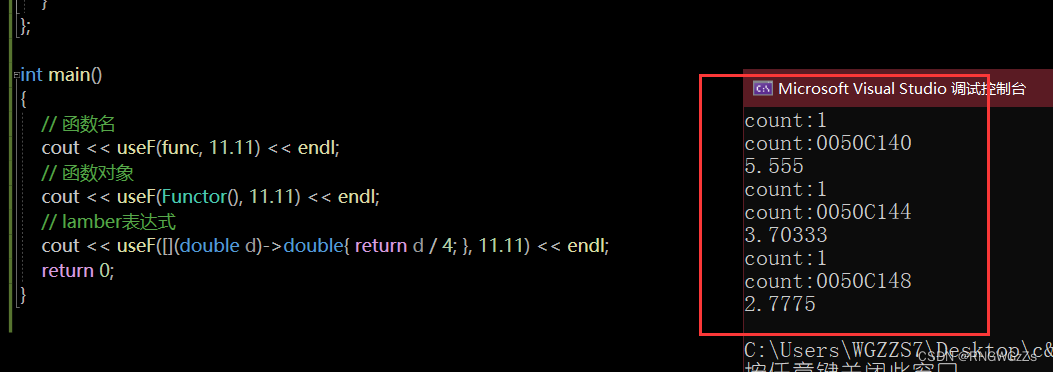### ①包装器的使用

``````int f1(int a, int b)
{
return a + b;
}

struct Functor1
{
public:
int operator() (int a, int b)
{
return a + b;
}
};

class Plus
{
public:
static int plusi(int a, int b)
{
return a + b;
}

double plusd(double a, double b)
{
return a + b;
}
};
#include<functional>

int main()
{
//包装器  function<Ret(Args...)>
//包装函数指针 f1
std::function<int(int, int)> ff1 = f1;
cout << f1(1, 2) << endl;
//包装 仿函数
std::function<int(int, int)> ff2 = Functor1();
cout << ff2(1, 2) << endl;
//lambda 表达式
auto f = [](int x, int y) {return x + y;};
std::function<int(int, int)> ff3 = f;
cout << f(1, 2) << endl;

//成员函数 的包装
//包装静态 函数
std::function<int(int, int)> pf = Plus::plusi;
cout << pf(1, 2) << endl;

//包装非静态              //this 找到调用函数
std::function<double(Plus,double, double)> ppf = &Plus::plusd; //成员函数 需要取地址
cout << ppf(Plus(), 1.1, 2.2) << endl;
//传Plus()匿名对象 主要是 this
}``````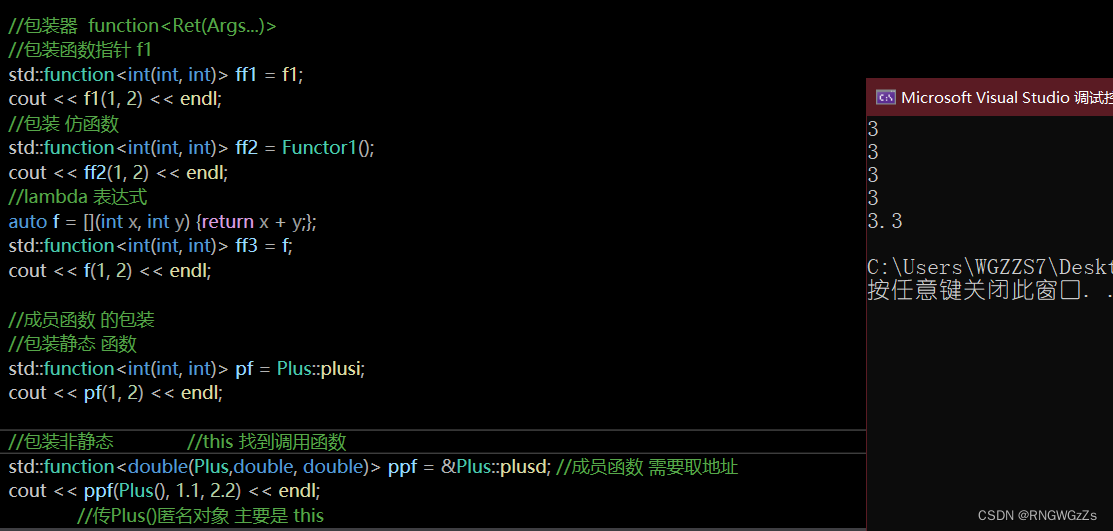``````	// 函数名
std::function<double(double)> f1 = func;
//cout << useF(func, 11.11) << endl;
cout << useF(f1, 11.11) << endl;
// 函数对象
std::function<double(double)> f2 = Functor();
//cout << useF(Functor(), 11.11) << endl;
cout << useF(f2, 11.11) << endl;

// lamber表达式
std::function<double(double)> f3 = [](double d)->double { return d / 4; };
//cout << useF([](double d)->double{ return d / 4; }, 11.11) << endl;
cout << useF(f3, 11.11) << endl;``````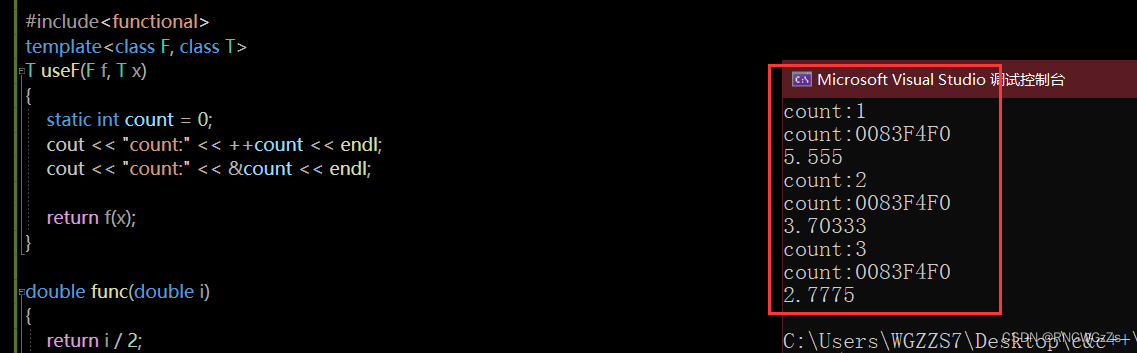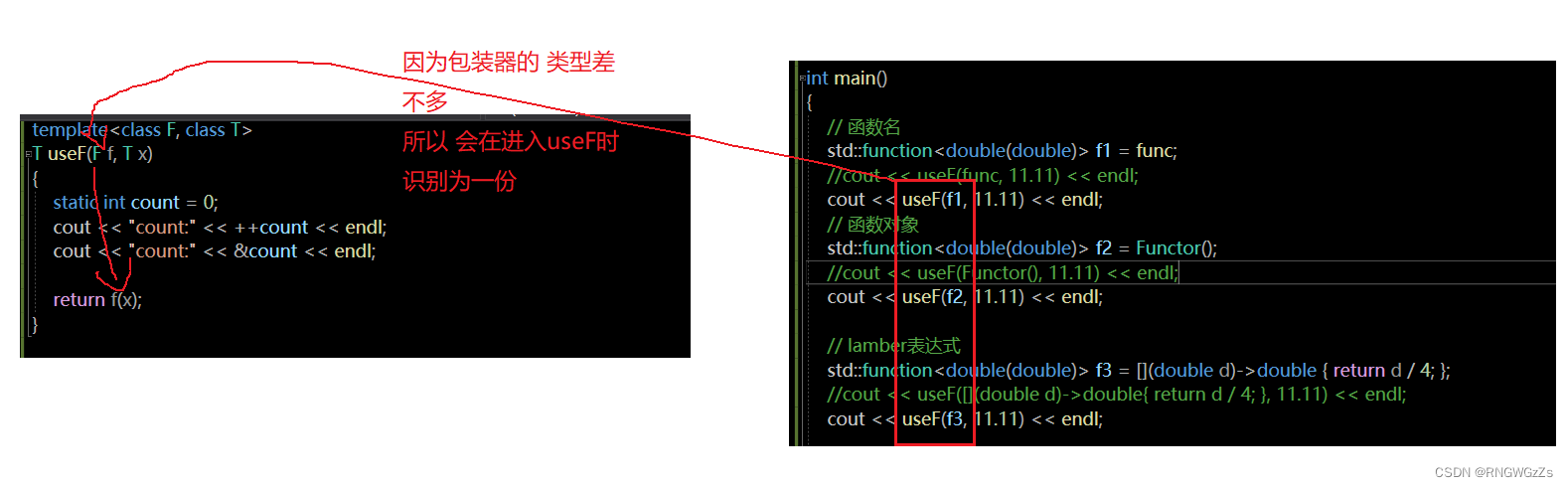lambda:语法层看不到类型(类型在底层)，lambda+uuid;

std::bind 调整可调用类型的参数

### ②bind使用

bind通常和 function 一起使用;

bind(可调用对象,....,placeholders::_1.placeholders::_2,placeholders...)

placeholders:命名空间 参数放的位置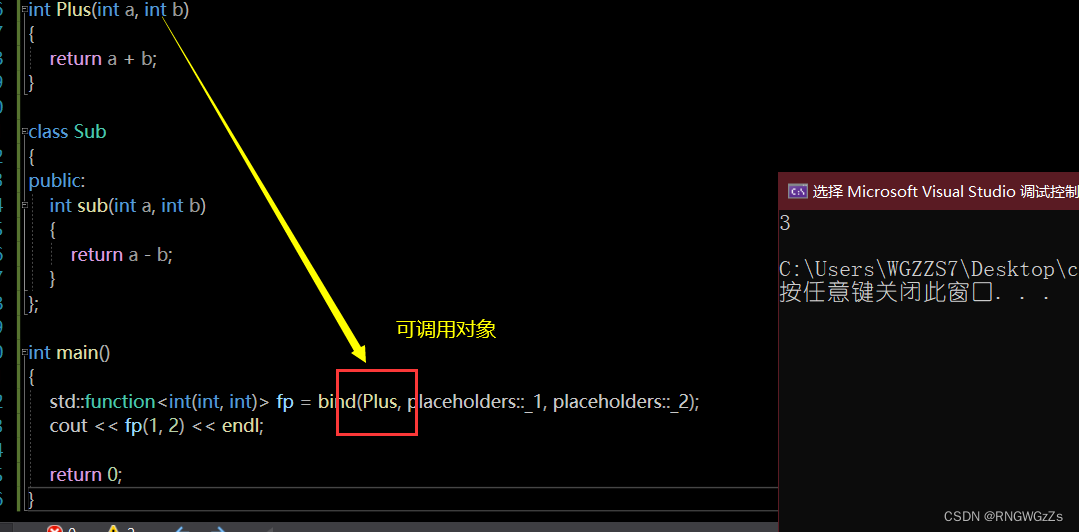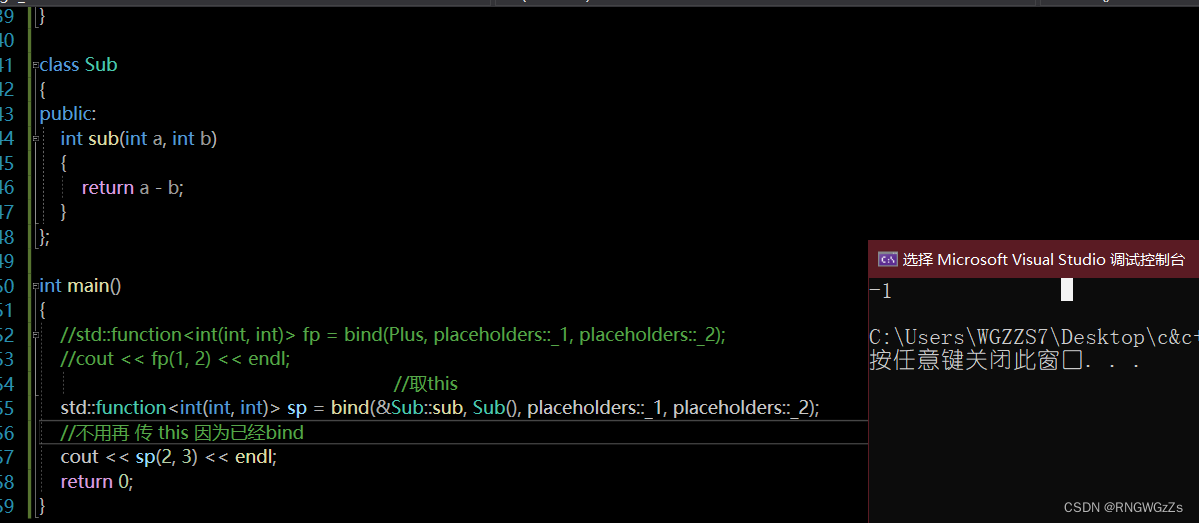C++11为了保障 线程库的可移植性(Linux的posix),对库进行了封装。有Linux多线程操作的基础,在使用上也更简单和容易上手。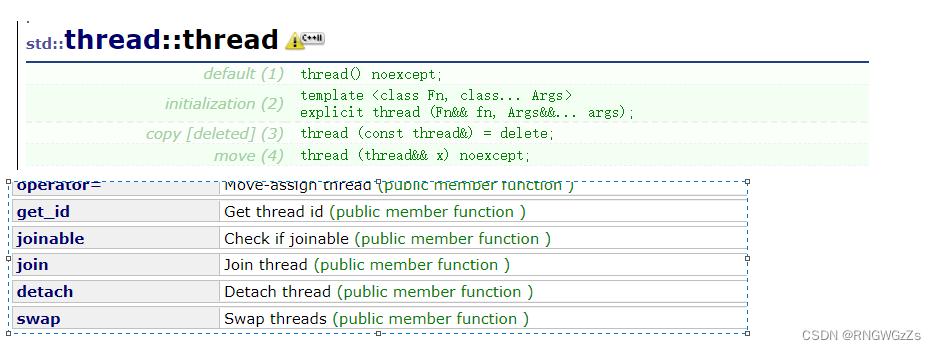### ①动态创建多个线程:

``````void f(int N)
{
for (int i = 0;i < N;++i)
{
cout << this_thread::get_id() << ":" << i << endl;
}
cout << endl;
}

int main()
{
int n;
cin >> n;

{
td = thread(f,10);  //拷贝构造被 禁止 但是支持了 移动赋值~
//1.cout<<td.get_id()<<endl;
}

{
td.join();
}
return 0;
}``````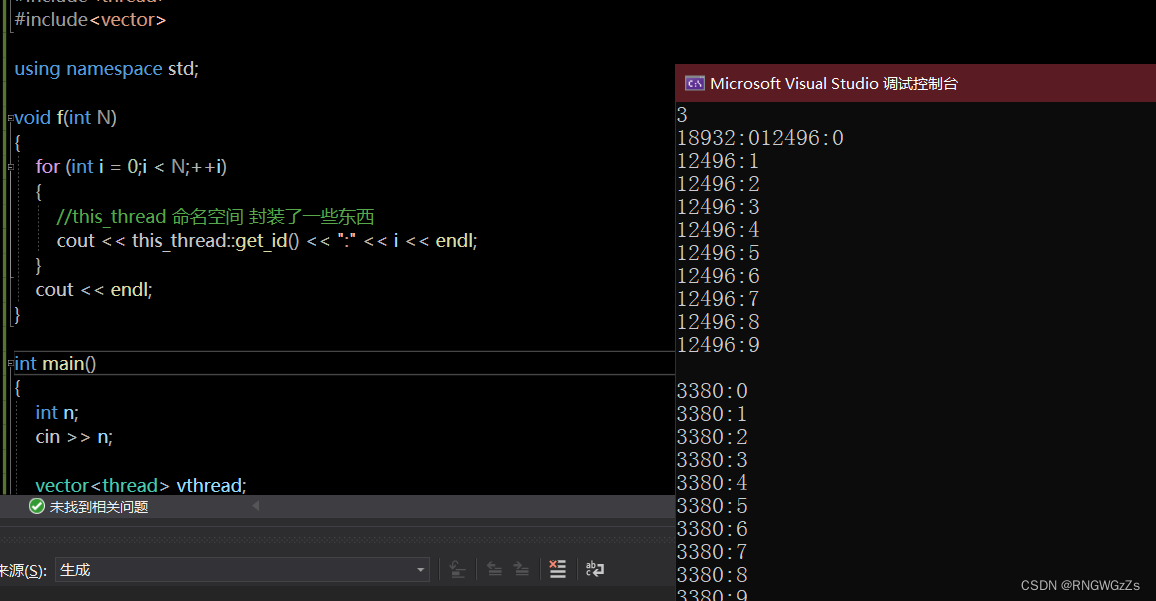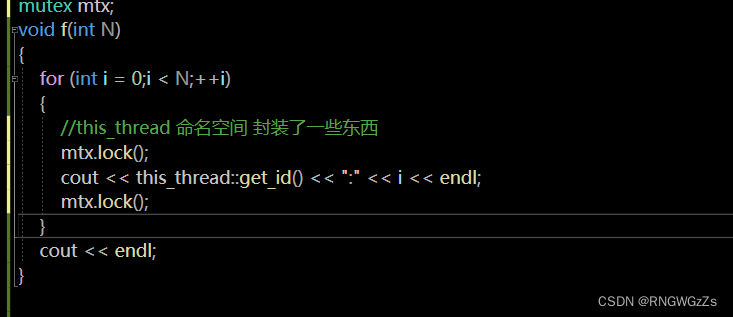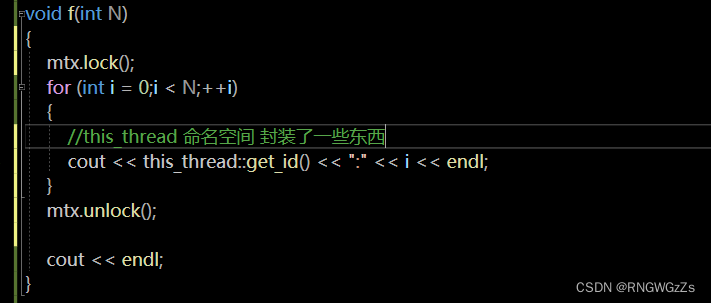### ②原子性atomic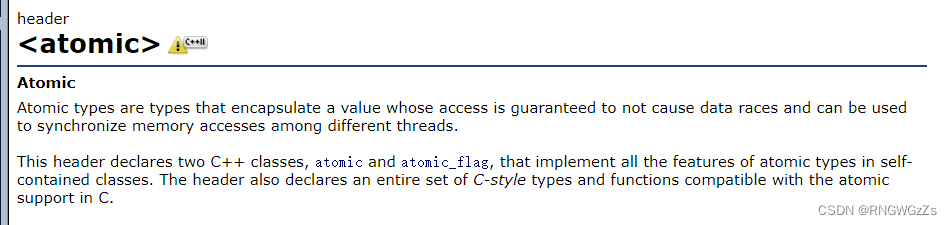``````atomic<int> x=0;
++x ; //此时++x 可以保证原子性

//非模板
atomic_int x=0; ``````

### ③unique_lock:``````void f()
{

std::unique_lock<mutex> ul(mtx);
......// 中间的代码是安全的

}``````

### 问题:如何实现两个线程,分别交替打印奇数、偶数？

只能保证互斥!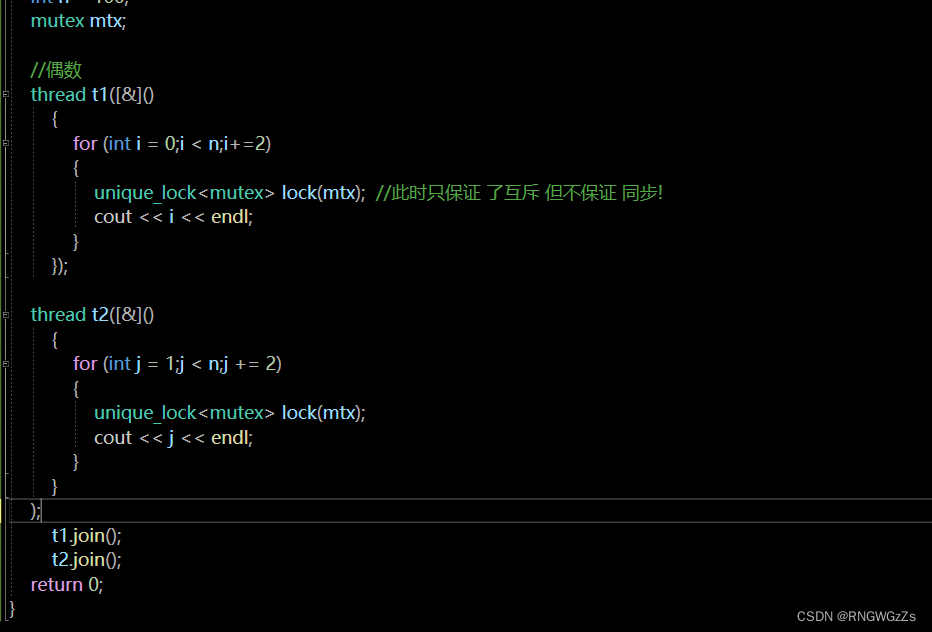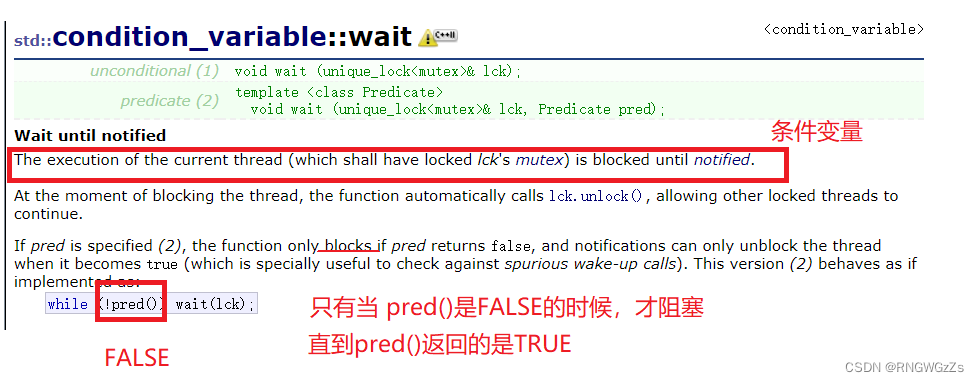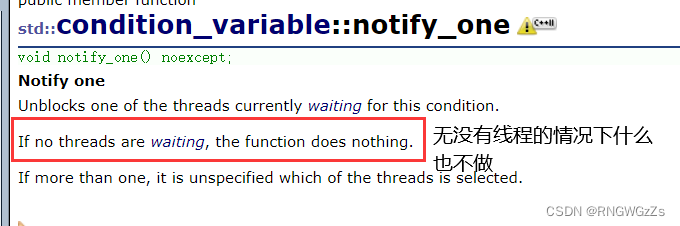``````int main()
{
int n = 100;
mutex mtx;
condition_variable cv;//条件变量
bool flag = true; //控制条件变量
//偶数
{
for (int i = 0;i < n;i+=2)
{
unique_lock<mutex> lock(mtx);  //此时只保证 了互斥 但不保证 同步!
cv.wait(lock, [&flag]()->bool {return flag;});
flag = false;
cout << i << endl;
cv.notify_one(); //唤醒
}
});

{
for (int j = 1;j < n;j += 2)
{
unique_lock<mutex> lock(mtx);
cv.wait(lock, [&flag]()->bool {return !flag;});
flag = true;
cout << j << endl;
cv.notify_one();
}
}
);

t1.join();
t2.join();
return 0;
}``````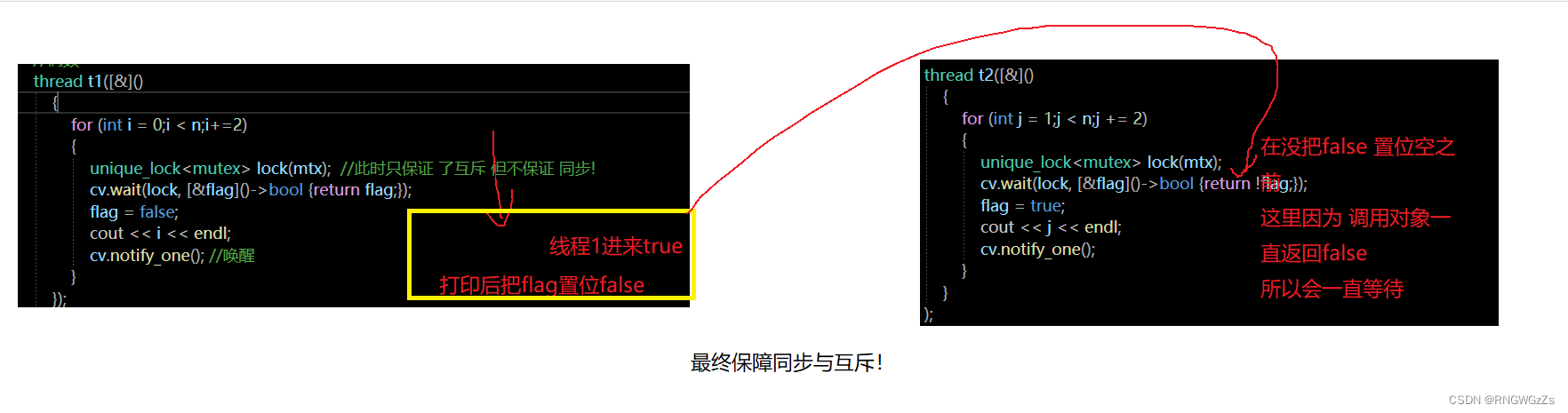# (二)设计模式

### (1)设计一个类 只能创建再堆上

``````class HeapOnly
{
public:
//static 不用this指针 可以外部突破类域进行访问
static HeapOnly* Create_Heap()
{
new HeapOnly();
}

private:
//为了只能再堆上开辟 所以仅需要把构造函数设为私有
HeapOnly()
{}

//为了防止 通过拷贝赋值 破坏规则
//C++98中对拷贝赋值的处理方式上
//只声明 不实现!
//但这种方式 有个缺陷，会在类外进行 实现
//HeapOnly(const HeapOnly&);

//C++11 因为引入了关键字
HeapOnly(const HeapOnly&) = delete;
//直接禁用掉了 这种可能
};

HeapOnly::HeapOnly(const HeapOnly&);

int main()
{
HeapOnly* p = HeapOnly::Create_Heap();

return 0;
}``````

### (2)在栈上开辟空间

``````//栈上开辟 主要就是 不使用new构造对象
class StackOnly
{
public:
StackOnly()
{}

private:
//C98 的方式 只声明 不实现
//void* operator new(size_t size);
//void operator delete(void* p);
void* operator new(size_t size)=delete;
void operator delete(void* p)=delete;
};

int main()
{
//如果局部没有 对new进行重写 则用全局new
//进行了 重载 就用本地new
//StackOnly st = new StackOnly;

//唯一的不足是 没有彻底禁止 在静态区创建
static StackOnly st;
return 0;
}``````

``````class StackOnly
{
public:
static StackOnly CreateObject()
{
return StackOnly();
}
private:
StackOnly() {}
};

int main()
{
//如果局部没有 对new进行重写 则用全局new
//进行了 重载 就用本地new
//StackOnly st = new StackOnly;

//唯一的不足是 没有彻底禁止 在静态区创建
StackOnly st = StackOnly::CreateObject();
return 0;
}``````

### (3)不能被继承

``````class NonInherit
{
public:
static NonInherit GetInstance()
{
return NonInherit();
}
private:
NonInherit()
{}
};

class	B :public NonInherit
{
public:

protected:
//.....
};``````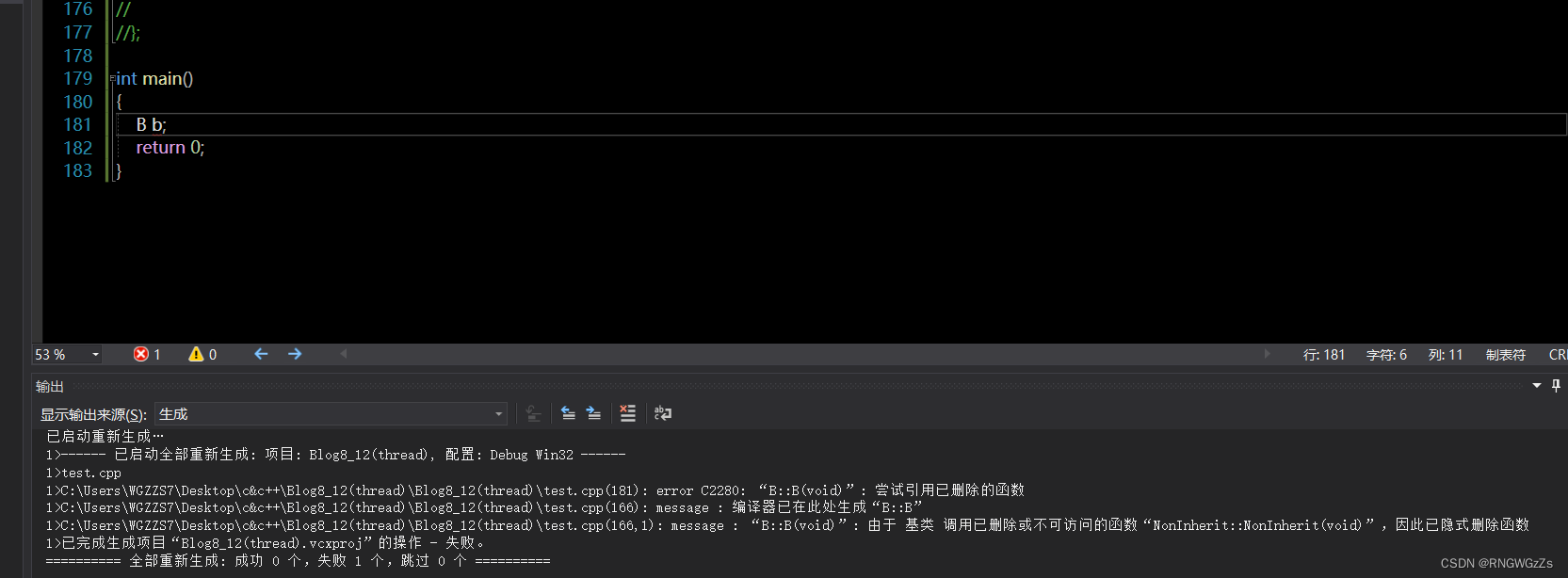缺陷在于,没有实例化的时候,编译是过的.因为实例化，子类才会去调用父类的构造函数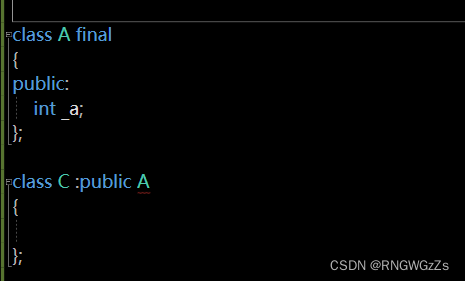## (1)单例模式

### ①饿汉模式

``````class Singleton
{
public:
static Singleton* GetInstance()
{
return _inst;
}
private:
//单例模式对象只能有一个
Singleton()
{}
//拷贝 和 赋值禁用
Singleton(const Singleton&) = delete;
Singleton& operator=(const Singleton&) = delete;
static Singleton* _inst; //唯一指向对象的指针

};
//在 main还没开始时 就已经进行 初始化了
Singleton* Singleton::_inst=new Singleton;``````

### ②懒汉模式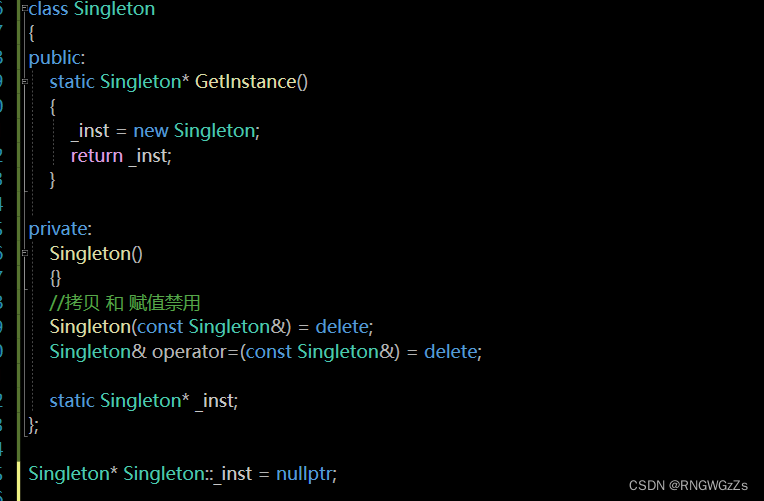懒汉模式的 不同点在于,_inst并不是在类外全局初始化.反观饿汉模式,则对static进行了初始化。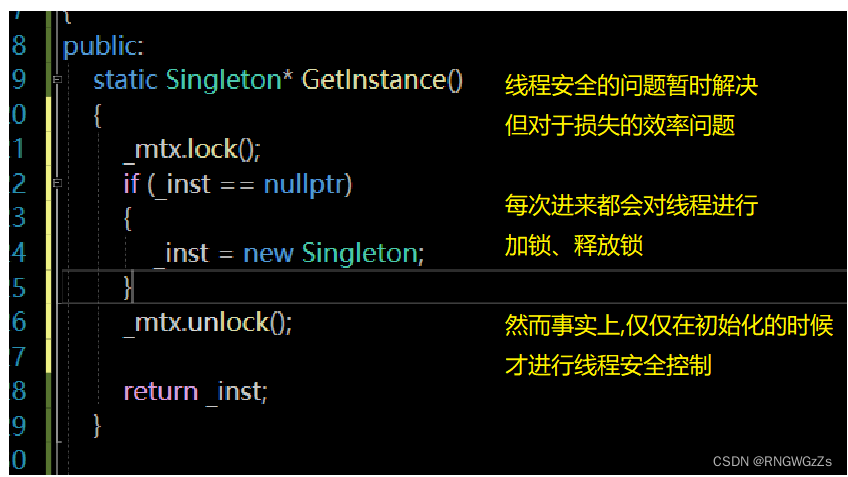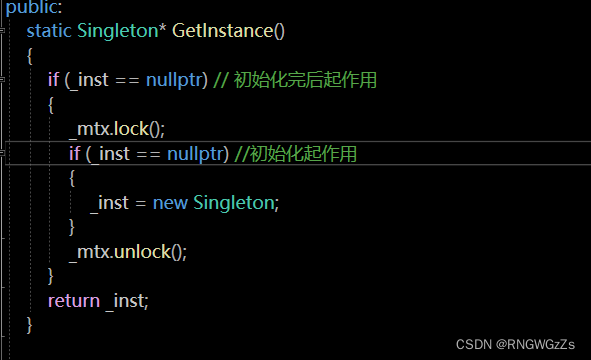因为对象是在堆上开辟的,也就必然牵涉到资源回收问题。

``````//懒汉模式
class Singleton
{
public:
static Singleton* GetInstance()
{
if (_inst == nullptr) // 初始化完后起作用
{
_mtx.lock();
if (_inst == nullptr) //初始化起作用
{
_inst = new Singleton;
}
_mtx.unlock();
}
return _inst;
}

private:
Singleton()
{}
//拷贝 和 赋值禁用
Singleton(const Singleton&) = delete;
Singleton& operator=(const Singleton&) = delete;

class CGarbo
{
public:
~CGarbo()
{
if (_inst)
{
delete _inst;
_inst = nullptr;
}
}
};

static Singleton* _inst;
static mutex _mtx;
static CGarbo _cg;
};

Singleton* Singleton::_inst = nullptr;
mutex Singleton::_mtx;
Singleton::CGarbo Singleton::_cg;``````

### ③其他版本的懒汉模式

``````class Singleton
{
public:
static Singleton* GetInstance()
{
//仅仅在这一次 会对inst进行初始化
static Singleton inst;

return &inst;
}

void Print()
{
cout << "Print()" << _a << endl;
}

private:
Singleton()
:_a(0)
{
// 假设单例类构造函数中，要做很多配置初始化
}

~Singleton()
{
// 程序结束时，需要处理一下，持久化保存一些数据
}

Singleton(const Singleton&) = delete;
Singleton& operator=(const Singleton&) = delete;
int _a;
};``````

### ④懒\饿汉模式的反思

1、如果单例对象构造函数(初始化)工作比较多，会导致程序启动慢,迟迟进不了入口main函数

2、如果有多个单例对象，他们之间有初始化依赖关系，饿汉模式也会有问题。

# 总结:

① 列表初始化的特性,是为了支持initializer_list。当已知数据内容,可以更方便对STL(vector、list、map)进行初始化赋值。

②final：修饰类 不可继承|修饰虚函数 不可重写:overrid：检查子类虚函数是否重写

③左值可以取地址,一般能修改。右值不可取地址,且不可修改!

④左值引用(T&) 可以引用左值 ，右值引用(T&&) 可以引用右值。

const 左值引用可以 引用右值；

⑤移动构造和移动赋值本质上是一种掠夺(转移)资源的手段，从而对深拷贝类,减少深拷贝,提高效率。

⑥万能引用能接收 右值+左值。右值每被引用,就会退化成 左值(forward<T>(x) 可以保持原属性)

⑦可变参数模板(Args ...);emplace系列的插入的效率,主要体现在,用参数包(args...)去构造

⑧lambda表达式的语法+使用。底层会识别为operator()

⑨包装器就是对 可调用对象的 返回值、参数可视化。bind通常与包装器(std::function<T(T,T)>)连用,调整可调用类型的参数。

(11)单例模式的两种方式:饿汉 懒汉